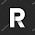## Maximum Product Subarray

Find the contiguous subarray within an array (containing at least one number) which has the largest product.

For example, given the array [2,3,-2,4],
the contiguous subarray [2,3] has the largest product = 6.

### Analysis:

Brutal force method will take O(n^2) time complexity, which is not acceptable.
Since it requires the "largest" product in an array, it is common to consider the DP(dynamic programming) method. And also since O(n^2) is NOT acceptable, there might be an O(n) solution.

So, given a whole scan , how to find the maximum product?
Note that, we need to consider two cases:
(1) negative numbers
Store the minimum product to handle the case that new element < 0. Because if current element < 0, the product of two negative numbers (new element, and minimum product before the new element) become positive.
(2) zeros
When meets zero, current max and min product become 0, new search is needed from the next element.

Therefore,  we can write down to function to store both + and - products:

max_product = max{A[i]*min_product (when A[i]<0),  A[i]*max_product (when A[i]>0),  A[i] (when 0 occurs before A[i]) }.

min_product = min{A[i]*min_product,  A[i]*max_product,  A[i] }.

Because current sequence might start from any element, to get the final result, we also need to store the max product after each iteration "res = max(res, maxp);".

### Code(C++):

class Solution {
public:
int maxProduct(int A[], int n) {
int res = A;
int maxp = A;
int minp = A;
for (int i=1;i<n;i++){
int tmpmax = maxp;
int tmpmin = minp;
maxp = max(max(tmpmax*A[i],tmpmin*A[i]),A[i]);
minp = min(min(tmpmax*A[i],tmpmin*A[i]),A[i]);
res = max(maxp,res);
}
return res;
}
};


### Code(Python):

class Solution:
# @param A, a list of integers
# @return an integer
def maxProduct(self, A):
res = A
minp = A
maxp = A
for a in A[1:]:
tmp_maxp = maxp
tmp_minp = minp
maxp = max(max(tmp_maxp*a, tmp_minp*a), a)
minp = min(min(tmp_maxp*a, tmp_minp*a), a)
res = max(res, maxp)
return res



1.2.